# C125 – Ulatrasonic Meter – Flowrate Calculation

## Description

This calculates the flowrate, applying corrections for the elastic distortion of the ultrasonic meter spool due pressure and thermal expansion.

DanielTM Ultrasonic Gas Flow Meters with Mark III Electronics – Reference, Installation, and Operations Manual

Kelton calculation reference C125

FLOCALC calculation reference F048

KIMS calculation reference K109

Kelton calculation C244 – Calibration Correction

## Options

### Flow velocity

• User Entered
• Calculated

Select based on whether flow velocity is an unknown.

### Juniorsonic correction

• Profile-effect correction

Check box if profile-effect correction is needed. This should only be applied to Juniorsonic meters.

### Calibration Correction

• None
• Before spool correction
• After spool correction

This option is used to specify whether there is a linearization applied to the flow rate. If linearization is applied to the raw volume flow rate then select before spool correction, if it is done after correction factors have been applied select after spool correction. The calibration correction is carried out according to C244 – Calibration Correction.

## Calculation

### Uncorrected volume flow rate

The uncorrected volume flow rate is calculated by: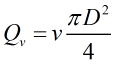Where v = Flow velocity D = Internal diameter

### Observed volume flow rate

The observed volume flow rate is calculated by: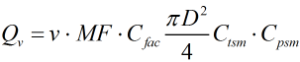Where v = Flow velocity MF = Meter Factor Cfac = C-factor D = Internal diameter Ctsm = Temperature correction factor Cpsm = Pressure correction factor

### Temperature correction factor

The temperature correction factor is calculated by: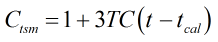Where TC = Temperature coefficient t = Temperature tcal = Calibration temperature

### Pressure correction factor

The pressure correction factor is calculated by: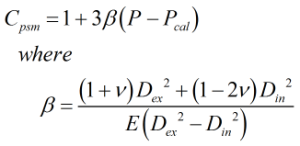Where β = Strain per unit stress P = Pressure Pcal = Calibration pressure E = Young’s modulus ν = Poisson’s ratio (note this is set to 0.3) Dex = External diameter Din = internal diameter

### Profile-effect correction

The profile-effect correction factor is for Juniorsonic meters and is calculated as follows: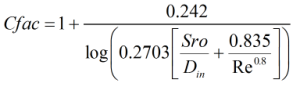Where Din = internal diameter Re = Reynolds number Sro = Spool wall roughness

Back